# Crystal Optics

Also found in: Wikipedia.

## Crystal optics

The study of the propagation of light, and associated phenomena, in crystalline solids. For a simple cubic crystal the atomic arrangement is such that in each direction through the crystal the crystal presents the same optical appearance. The atoms in anisotropic crystals are closer together in some planes through the material than in others. In anisotropic crystals the optical characteristics are different in different directions. In classical physics the progress of an electromagnetic wave through a material involves the periodic displacement of electrons. In anisotropic substances the forces resisting these displacements depend on the displacement direction. Thus the velocity of a light wave is different in different directions and for different states of polarization. The absorption of the wave may also be different in different directions. See Dichroism, Trichroism

In an isotropic medium the light from a point source spreads out in a spherical shell. The light from a point source embedded in an anisotropic crystal spreads out in two wave surfaces, one of which travels at a faster rate than the other. The polarization of the light varies from point to point over each wave surface, and in any particular direction from the source the polarization of the two surfaces is opposite. The characteristics of these surfaces can be determined experimentally by making measurements on a given crystal.

In the most general case of a transparent anisotropic medium, the dielectric constant is different along each of three orthogonal axes. This means that when the light vector is oriented along each direction, the velocity of light is different. One method for calculating the behavior of a transparent anisotropic material is through the use of the index ellipsoid, also called the reciprocal ellipsoid, optical indicatrix, or ellipsoid of wave normals. This is the surface obtained by plotting the value of the refractive index in each principal direction for a linearly polarized light vector lying in that direction (see illustration). The different indices of refraction, or wave velocities associated with a given propagation direction, are then given by sections through the origin of the coordinates in which the index ellipsoid is drawn. These sections are ellipses, and the major and minor axes of the ellipse represent the fast and slow axes for light proceeding along the normal to the plane of the ellipse. The length of the axes represents the refractive indices for the fast and slow wave, respectively. The most asymmetric type of ellipsoid has three unequal axes. It is a general rule in crystallography that no property of a crystal will have less symmetry than the class in which the crystal belongs.

Accordingly, there are many crystals which, for example, have four- or sixfold rotation symmetry about an axis, and for these the index ellipsoid cannot have three unequal axes but is an ellipsoid of revolution. In such a crystal, light will be propagated along this axis as though the crystal were isotropic, and the velocity of propagation will be independent of the state of polarization. The section of the index ellipsoid at right angles to this direction is a circle. Such crystals are called uniaxial and the mathematics of their optical behavior is relatively straightforward.

In crystals of low symmetry the index ellipsoid has three unequal axes. These crystals are termed biaxial and have two directions along which the wave velocity is independent of the polarization direction. These correspond to the two sections of the ellipsoid which are circular. See Crystallography

The normal to a plane wavefront moves with the phase velocity. The Huygens wavelet, which is the light moving out from a point disturbance, will propagate with a ray velocity. Just as the index ellipsoid can be used to compute the phase or wave velocity, so can a ray ellipsoid be used to calculate the ray velocity. The length of the axes of this ellipsoid is given by the velocity of the linearly polarized ray whose electric vector lies in the axis direction. See Phase velocity

The refraction of a light ray on passing through the surface of an anisotropic uniaxial crystal can be calculated with Huygens wavelets in the same manner as in an isotropic material. For the ellipsoidal wavelet this results in an optical behavior which is completely different from that normally associated with refraction. The ray associated with this behavior is termed the extraordinary ray. At a crystal surface where the optic axis is inclined at an angle, a ray of unpolarized light incident normally on the surface is split into two beams: the ordinary ray, which proceeds through the surface without deviation; and the extraordinary ray, which is deviated by an angle determined by a line drawn from the center of one of the Huygens ellipsoidal wavelets to the point at which the ellipsoid is tangent to a line parallel to the surface. The two beams are oppositely linearly polarized.

## Crystal Optics

an area between optics and crystal physics, encompassing the study of the propagation of light in crystals. Phenomena characteristic of crystals that are studied in crystal optics include double refraction, polarization of light, rotation of the plane of polarization, and pleochroism.

The phenomenon of double refraction was first observed in crystals of Iceland spar by the Danish scientist E. Bartholin in 1669. This date is considered the beginning of crystal optics. Problems of the absorption and emission of light by crystals are studied in crystal spectroscopy. The effect of electric and magnetic fields on the optical properties of crystals is studied in electro-optics and magneto-optics, which are based on the fundamental laws of crystal optics.

Since the lattice constant (of the order of 10 angstroms [Å]) is much smaller than the wavelength of visible light (4,000–7,000 Å), a crystal may be regarded as a homogeneous but anisotropic medium. The optical anisotropy of crystals is caused by the anisotropy of the force field of particle interaction. The nature of the field is related to crystal symmetry. All crystals, except crystals of the cubic system, are optically anisotropic.

The optical anisotropy of transparent nonmagnetic crystals is due to the anisotropy of the dielectric constant c. In isotropic mediums the electric induction vector D is related to the electric field vector E by the equation D = ∊E, where € is a scalar quantity that depends on the frequency in the case of alternating fields. Thus, the vectors D and E have the same direction in isotropic mediums. In crystals the directions of the vectors D and E do not coincide, and the relationship between D and E is more complex, since the dielectric constant e, a tensor quantity, depends on direction in the crystal. A result of this is the ob-served anisotropy of the optical properties of crystals, particularly the dependence on direction of the rate of propagation v of waves and of the index of refraction n. The dependence of the components of the dielectric constant tensor on wave frequency explains the dispersion of the optical properties of crystals.

The dependence on direction of the dielectric constant —and, consequently, of the index of refraction n—can be represented graphically. If radius vectors r with magnitudes r = n = √є, where ∊ is the dielectric constant in direction r, are drawn in all directions from an arbitrary point O of the crystal, then the ends of the vectors r lie on the surface of an ellipsoid, called the optical indicatrix or index ellipsoid (Figure 1). The axes of symmetry of the ellipsoid define the three mutually perpendicular principal directions in the crystal. In a rectangular Cartesian coordinate system, whose axes coincide with the principal directions, the equation of the optical indicatrix is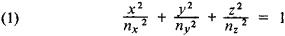where nx, ny, and nz are the values of n along the principal directions (principal values of the tensor of the dielectric constant and of n). The optical axis of the crystal is a straight line that passes through a given point O of the crystal at right angles to the plane of a circular section of the optical indicatrix.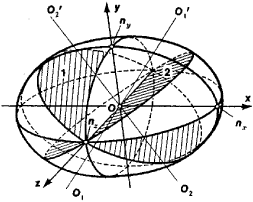Figure 1. Optical indicatrix of a biaxial crystal, a triaxial ellipsoid: (ox), (oy), and (oz) axes of symmetry of the ellipsoid, called the principal axes of the indicatrix; (nx), (ny), and (nz) indexes of refraction along the principal axes; (1) and (2) two circular sections of the ellipsoid; (O1,O1) and (O2O2) optical axes of the crystal

In the case of cubic crystals, which are optically isotropic, ∊ is independent of direction, and the optical indicatrix becomes a sphere with radius r = n = √є. In crystals of intermediate systems (trigonal, tetragonal, and hexagonal), one of the principal directions coincides with the main axis of symmetry of the crystal. The optical indicatrix in such crystals is an ellipsoid of revolution, and the crystals have only one optical axis, which coincides with the axis of rotation of the ellipsoid. Such crystals are called uniaxial. A uniaxial crystal is called optically positive (+) if its optical axis coincides with the long axis of the optical indicatrix (the ellipsoid is elongated along the axis of rotation) and optically negative (−) if the ellipsoid is compressed along the axis of rotation. Crystals of the lower systems (rhombic, monoclinic, and triclinic) are called biaxial. Their optical indicatrix is a triaxial ellipsoid, which has two circular sections and two optical axes (Figure 1).

Because of the noncoincidence of the direction of the vectors D and E, a polarized, plane monochromatic wave in a crystal is characterized by two triads of mutually perpendicular vectors D, H, v and E, H, v′ (Figure 2). The velocity v′ coincides in direction with the Poynting vector S and is equal to the rate of energy transfer by the wave. It is called the ray velocity of the wave. The velocity v is called the normal velocity of the wave. It is equal to the rate of propagation of the phase and the wave front in the direction of the normal vector N to the front. The quantities v and v′ are connected by the relation v′ = v/cos α, where α is the angle between the vectors D and E.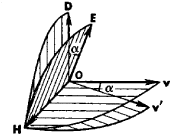Figure 2

The normal and ray velocities of the wave are determined from the Fresnel equation, which is the basic equation of crystal optics: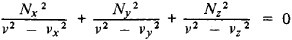Here Nx, Ny, and Nz are projections of the vector normal N onto the principal directions of the crystal; vx = c/ny, vy = c/nz are the principal phase velocities of the wave; c is the velocity of light in a vacuum; and nx, ny, nz are the principal indexes of refraction of the crystal.

Since the Fresnel equation is quadratic with respect to v, for any direction N there are two values of the normal velocity, v1 and v2, which coincide only in the direction of the optical axes of the crystal. If vectors of the corresponding normal velocities v are plotted from a point O in all directions N, then the ends of the vectors lie on a surface called the normal surface. It is a double-sheeted surface, each sheet of which corresponds to one of the values of v for a given direction N. In the case of a uniaxial crystal, one surface is a sphere and the other is an ovaloid, which touches the sphere at its two points of intersection with the optical axis. In the case of a biaxial crystal, the surfaces intersect at four points located on the two optical axes (binormals).

Similarly, the geometric locus of points at a distance v′ from a point O is called the ray surface, or wave surface. It is the wave surface for waves propagating within the crystal from a point source located at point O. This is also a double-sheeted surface. In uniaxial crystals, one surface is a sphere and the other is an ellipsoid of revolution about the optical axis Oz. The sphere and the ellipsoid touch in the points of their intersection with the optical axis. In positive crystals the ellipsoid is inscribed within the sphere (Figure 3,a), whereas in negative crystals the sphere is inscribed within the ellipsoid (Figure 3,b). In biaxial crystals, the surfaces intersect at four points located in pairs on two straight lines that intersect at point O (biradials).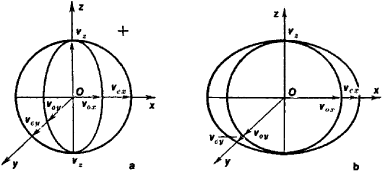Figure 3. Ray surfaces of uniaxial crystals: (a) positive, (b) negative, (OZ) optical axis of the crystal, (v0) and (vc) phase velocities of two waves propagating in the crystals

Thus, two plane waves that are polarized in two mutually perpendicular planes may propagate in an arbitrary direction N in crystals. The directions of the vectors D1 and D2 of the waves coincide with the axes of the ellipse resulting from intersection of the optical indicatrix with the plane perpendicular to N and passing through the point O. The normal velocities of the waves are v1 = c/n1 and v2 = c/n2. The vectors E1 and E2 of the waves are also located on two perpendicular planes, and there are two corresponding ray vectors, Si and S2, and two values of the ray velocities, v1 = v1′ cos α and v2 = v2′ cos α. Similarly, for a given direction of the ray S there are two possible directions for the vector E (E1E2), corresponding to the two values of the ray velocity, v1′ and v2′.

The dependence of the ray velocity of a plane wave propagating in a crystal on the direction of propagation and the nature of polarization of the wave leads to the splitting of light rays in crystals. In a uniaxial crystal, one of the refracted rays obeys the usual laws of refraction and is therefore called the ordinary ray, whereas the other ray does not (it does not lie in the plane of incidence) and is called the extraordinary ray. In a biaxial crystal, both rays are extraordinary.

During propagation in a crystal, the two waves arising upon refraction acquire a path difference because of differences in the indexes of refraction and the geometric path, although they re-main coherent. A polarization device may be used to bring the directions of oscillation of the waves emerging from the crystal into the same plane and to observe their interference. Interference of linearly polarized white light is manifested as coloration of the crystal, which depends on the path difference acquired by the beam. Characteristic interference patterns (”conoscopic patterns”) whose shape depends on the orientation of the crystal may sometimes be observed.

In crystals of some symmetry classes, rotation of the plane of polarization is possible in addition to double refraction. Two elliptically polarized waves (with opposite senses of rotation), each with its own index of refraction, may propagate in each direction in such crystals. The polarization is circular only in the direction of the optical axis, which leads to rotation of the plane of polarization of linearly polarized light incident on the crystal.

In the case of strongly absorbing crystals, a linearly polarized wave propagating in the crystal is split into two elliptically polarized waves with the same sense of rotation. Such crystals exhibit different absorption of waves with different polarization.

Each crystal has its own characteristic set of optical proper-ties, which can be used for identification. The most important properties for uniaxial crystals are the indexes of refraction n0 of the ordinary wave and ne of the extraordinary wave and the difference Δn between them (magnitude of the double refraction), as well as the dependence of the characteristics on the wave-length (various types of dispersion). Biaxial crystals are characterized by a more complex set of properties. In applied crystal optics, which is concerned with analysis of minerals and rocks, various methods for measuring these quantities were developed for various preparations of minerals in the form of powders and thin plates (sections). The principal methods are the immersion method, for determining the index of refraction using special liquids or melts with known indexes of refraction, and Fedorov’s method, for the determination of the orientation of the indicatrix, using a microscope stage that rotates the crystal about various axes. Most crystal-optics measurements are performed with a polarization microscope. There are reference books containing collected information on the optical properties of most known minerals.

The methods of crystal optics are of great importance in physics research (for example, in the generation of polarized light and analysis of elliptically polarized light; in various instruments for controlling beams of light) and in chemical engineering (analysis of materials; optical activity).

### REFERENCES

Born, M., and E. Wolf. Osnovy optiki. Moscow, 1970. (Translated from English.)
Landsberg, G. S. Optika, 4th ed. Moscow, 1957. (Obshchii kurs fiziki, vol. 3.)
Fedorov, F. I. Optika anizotropnykh sred. Minsk, 1958.
Shubnikov, A. V. Osnovy opticheskoi kristallografii. Moscow, 1958.
Beliankin, D. S., and V. P. Petrov. Kristallooptika, 4th ed. Moscow, 1951.
Tatarskii, V. B. Kristallooptika i immersionnyi metod issledovaniia mineralov. Moscow, 1965.
Ditchburn, R. Fizicheskaia optika. Moscow, 1965. (Translated from English.)

V. B. TATARSKII and B. N. GRECHUSHNIKOV

## crystal optics

[¦krist·əl ′äp·tiks]
(optics)
The study of the propagation of light, and associated phenomena, in crystalline solids.
References in periodicals archive ?
Anomalous crystal optics of heterogeneous crystals, Crystallography Reports 50(2): 297-307.
He bridges from the theory of guided wave and crystal optics, which is well covered in several textbooks, to the practice of lithium niobate device design and application, which is typically only described in the technical literature.
It uses patented Doubly Curved Crystal optics that are claimed to help deliver best-in-class limits of detection that allow users to conserve time and money by decreasing the number of inconclusive samples that require validation by off-site laboratory methods.
Berry, "Conical diffraction: Hamilton's diabolical point at the heart of crystal optics," in Progress in Optics, E.

Site: Follow: Share:
Open / Close